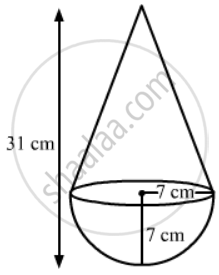Advertisement Remove all ads

# A Toy is in the Form of a Cone Mounted on a Hemisphere of Common Base Radius 7 Cm. the Total Height of the Toy is 31 Cm. Find the Total Surface Area of the Toy. - Mathematics

Sum

A toy is in the form of a cone mounted on a hemisphere of common base radius 7 cm. The total height of the toy is 31 cm. Find the total surface area of the toy.

Advertisement Remove all ads

#### SolutionRadius of the common base of the hemisphere and cone = 7 cm

Curved surface area of the hemisphere = 2pir^2 = 2xx22/7xx7xx7 = 308 cm2

"Height of the cone = Total height - Radius =31 - 7 =24 cm"

"Curved surface area of the cone" = pirl = pirlsqrt(r^2 + h^2) = 22/7 xx 7sqrt(49 + 576)=22sqrt(625) = 22xx25 = 550 cm2

Total surface area of the toy = 550 + 308 = 858 Cm2

Is there an error in this question or solution?
Advertisement Remove all ads

#### APPEARS IN

RS Aggarwal Secondary School Class 10 Maths
Chapter 19 Volume and Surface Area of Solids
Exercise 19A | Q 14 | Page 875
Advertisement Remove all ads
Advertisement Remove all ads
Share
Notifications

View all notifications

Forgot password?